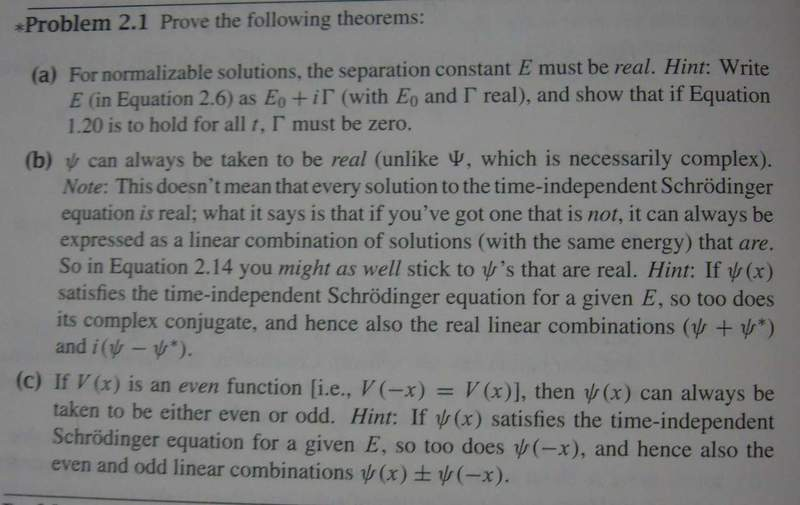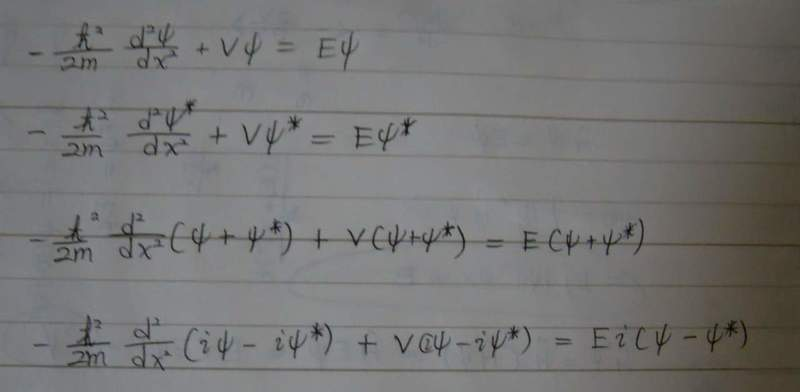# Wave function

## Homework StatementI don't know how to prove b.)

## The Attempt at a SolutionWhat can I do after writing these 4 eqtn?

#### Attachments

You're almost there. What do you know about a linear combination of solutions to a differential equation? What does that say about each independent solution?

You're almost there. What do you know about a linear combination of solutions to a differential equation? What does that say about each independent solution?

In fact that is the hint.
So can you also explain the hint?

And I still don't know how to get the answer:(

I'm asking it in a different way to get you to think about it. Do you know what a linear combination is? (If not then say so and I'll explain it). Have you taken a course in differential equations?

If both elements in the linear combination are individually solutions to the differential equation then is it also true that the two elements combined in a linear combination is then a solution?

Yes, I have taken courses about that and I know how to solve first and second linear differential eqtn.
However, all I know is how to calculate. I am not fully understand the meaning behind it.

Do you mean that I need to prove the solution for fourth eqtn is real.

i.e. $i(\psi-\psi*)$ is real?

ideasrule
Homework Helper
A differential equation is linear if none of the terms are being squared, cubed, square rooted, etc. Schrodinger's equation is linear because the wavefunction isn't being raised to any power, and neither is its second derivative.

For a linear system of equations (not necessarily differential equations), if you know that A and B are both solutions, all linear combinations of A and B must also be solutions. Here, you need to prove that if ψ is a solution to Schrodinger's equation, so is ψ*. Then you'll know that ψ+ψ* and i(ψ−ψ∗) are also solutions to Schrodinger's equation. But these are both real, which completes the proof.

A differential equation is linear if none of the terms are being squared, cubed, square rooted, etc. Schrodinger's equation is linear because the wavefunction isn't being raised to any power, and neither is its second derivative.

For a linear system of equations (not necessarily differential equations), if you know that A and B are both solutions, all linear combinations of A and B must also be solutions. Here, you need to prove that if ψ is a solution to Schrodinger's equation, so is ψ*. Then you'll know that ψ+ψ* and i(ψ−ψ∗) are also solutions to Schrodinger's equation. But these are both real, which completes the proof.

I wonder if i(ψ−ψ∗) is real, because it has a " i " in front of it.

vela
Staff Emeritus
Homework Helper
Let $\psi(x) = u(x) + i v(x)$ where u(x) and v(x) are real functions. Then $\psi^*(x) = u(x) - i v(x)$.

What are $\psi(x)+\psi^*(x)$ and $i(\psi(x)-\psi^*(x))$ equal to?

Let $\psi(x) = u(x) + i v(x)$ where u(x) and v(x) are real functions. Then $\psi^*(x) = u(x) - i v(x)$.

What are $\psi(x)+\psi^*(x)$ and $i(\psi(x)-\psi^*(x))$ equal to?

Oh! Thanks so much!!
Why I am so stupid that I didn't try to let $\psi^*(x) = u(x) - i v(x)$.Let $\psi(x) = u(x) + i v(x)$ where u(x) and v(x) are real functions. Then $\psi^*(x) = u(x) - i v(x)$.

What are $\psi(x)+\psi^*(x)$ and $i(\psi(x)-\psi^*(x))$ equal to?
Wait a minute, it is easy to solve because of the hint.
How about without the hint? How can I get the fourth eqtn?

Can I obtain it like this?
By mutiplying $i$ on eqtn 1 and 2, implys that $i\psi(x)$ and $i\psi(x)^*$ are soln to the eqtn.

By subtracting them get the fourth eqtn.

Am I right?

Here's my take on it:

Given

$$-\frac{{\hbar}^{2}}{2m}\frac{d^{2}{\psi}}{dx^{2}} + V{\psi} = E{\psi}$$

Taking the complex conjugate:

$$-\frac{{\hbar}^{2}}{2m}\frac{d^{2}{\psi}^{*}}{dx^{2}} + V{\psi}^{*} = E{\psi}^{*}$$

It's important to note that both V and E are real.

If we let ${\psi}_{1}$ and ${\psi}_{2}$ satisfy the first equation then so does some linear combination of them ${\psi}_{3}$ where:
$${\psi}_{3} = c_{1}{\psi}_{1} + c_{2}{\psi}_{2}$$

If we were to sub ${\psi}_{3}$ into the first equation we would expand out, rearrange blah blah blah simplify back down to the first equation again just with ${\psi}_{3}$ in place of ${\psi}$

So there we have proved that a linear combination will satisfy the equation. We also know that both ${\psi}$ and ${\psi}^{*}$ are independent solutions.

The linear combinations:
$${\psi} + {\psi}^{*}$$
and
$$i({\psi} - {\psi}^{*})$$
are solutions and are also real.

Thus we can conclude that from any complex solution we can construct two real solutions.

We can write:

$${\psi} = \frac{1}{2}[({\psi} + {\psi}^{*}) -i( i({\psi} - {\psi}^{*}))]$$
which we can simplify down to just ${\psi}$ we have thus proven that any ${\psi}$ can be written as a linear combination of two real solutions.